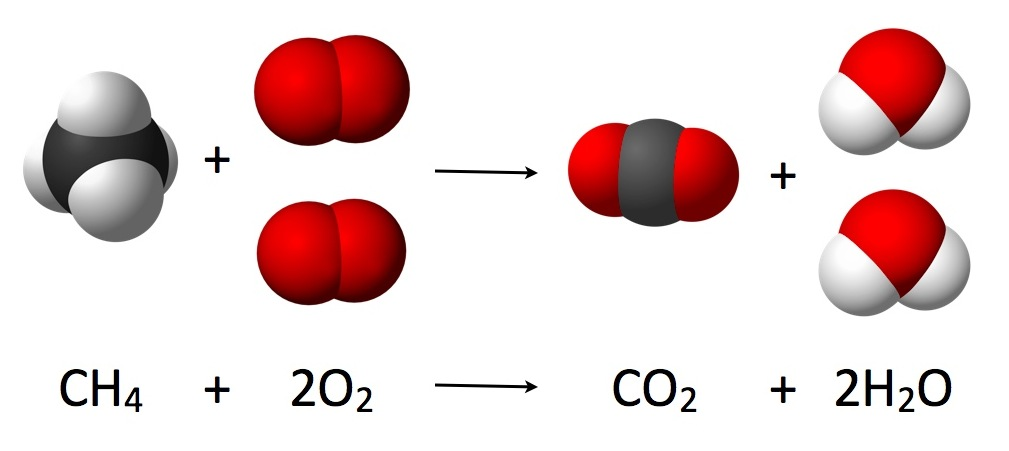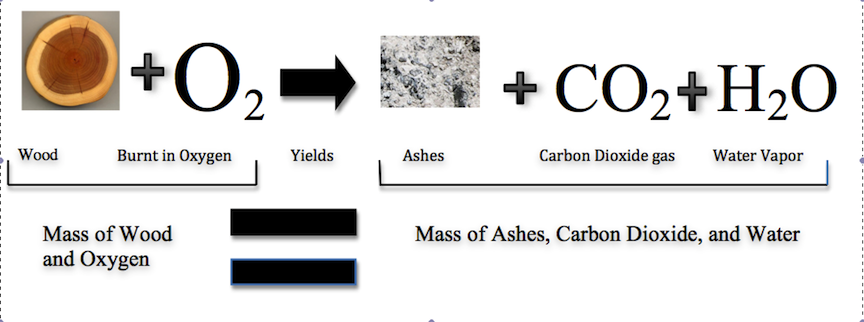Swati Talwar

HS-PS1-1

# The Law Of Conservation Of Mass Study Guide

The law of conservation of mass states that mass in an isolated system is neither created nor even destroyed by any chemical reaction or physical transformation.

# Introduction

The ancient Greeks first proposed the idea that the total amount of mass in the universe should be taken as constant. However, it was Antione Lavoisier who was among the first to have actually described the law conservation of mass as a fundamental principle of physics in 1789. The law states that even if the mass undergoes chemical reactions or physical transformations, it remains conserved; i.e., it is neither destroyed nor created. In a chemical reaction, the mass of the reactant would be equivalent to the mass of the product.Source

This law was then later amended by Einstein in the law of conservation of mass and energy.

## Law Of Conservation Of Mass Definition

Imagine you are in a sealed room, and there is no way that anything could go out or even enter. Now you light a candle, and after some time, you notice that some of the wax has melted and the candle seems to have partially vanished! Where did it go? Can the wax really disappear?

The Law of conservation of mass definition states that within a closed system, the mass within the system cannot get destroyed or change over time. Let us take the example we have discussed again. While the wax in the candle is no longer present in its original form, it is there in the room, albeit in a different form!

Therefore, to remember the law of conservation of mass in a simplified way, it could be said that the mass of the given reactants must be the same as that of the products. The law of conservation of mass is a useful concept in chemistry as the energy produced or consumed in any general chemical reaction accounts for a minute amount of mass.

We can now deduce that the atoms that are involved in any chemical reaction ultimately remain unchanged, even if in another form. This helps us present chemical equations as a balanced equation where the number of moles of any element is the same on both sides.

## Importance Of Law Of Conservation Of Mass In Chemistry

This law is crucial in understanding and production of various chemical reactions. If researchers know the identities alongside the quantities of the reactants for any particular reaction, then they could predict what the product amount would be. This law can be easily applied in laboratory practices.

## Examples Of Application Of Law Of Conservation Of Mass

Combustion process like the burning of wood follows this law which involves water vapor, carbon dioxide, and ashes.Source

To get water whose molecular weight would be 10, Hydrogen with 2 molecular weight and oxygen with 8 molecular weight undergoes reaction; therefore, conserving mass.

# Conclusion

• To understand the law of conservation of mass in a simplified way, the mass of the reactants must be equal to the mass of the products.

• It is useful in doing various calculations.

## FAQ

1. What Is The Law Of Conservation Of Mass Short Answer?

In short, the mass of the reactants must be equal to the mass of the products.

2. What Is The Best Example Of Law Of Conservation Of Mass?

A good example of the law of conservation of mass would be the combustion process.

1. Law of Conservation of Mass: https://courses.lumenlearning.com/introchem/chapter/the-law-of-conservation-of-mass/. Accessed 8th March 2022.
2. Law of Conservation of Mass: https://byjus.com/physics/law-of-conservation-of-mass/.Accessed 8th March 2022.
3. Definition of Law of Conservation of Mass:https://www.thoughtco.com/definition-of-conservation-of-mass-law-604412.Accessed 8th March 2022
4. Law of Conservation of Mass:https://study.com/academy/lesson/the-law-of-conservation-of-mass-definition-equation-examples.html.Accessed 8th March 2022.